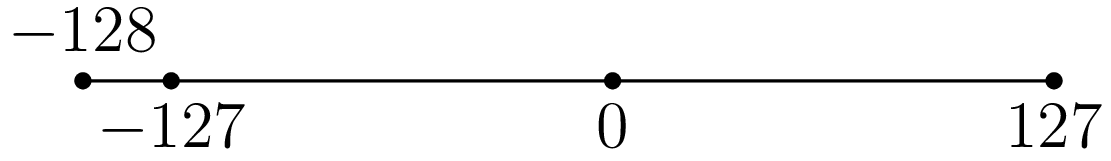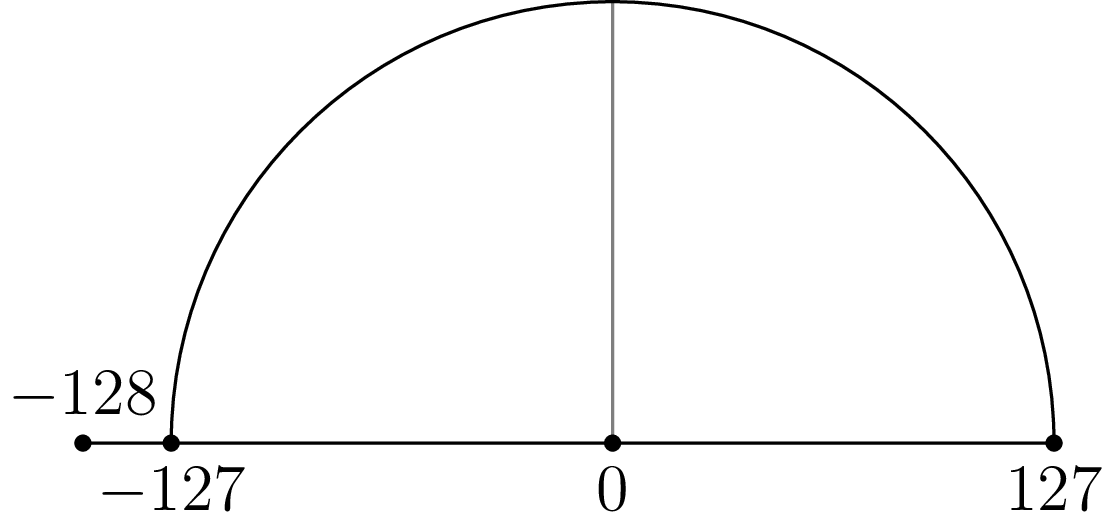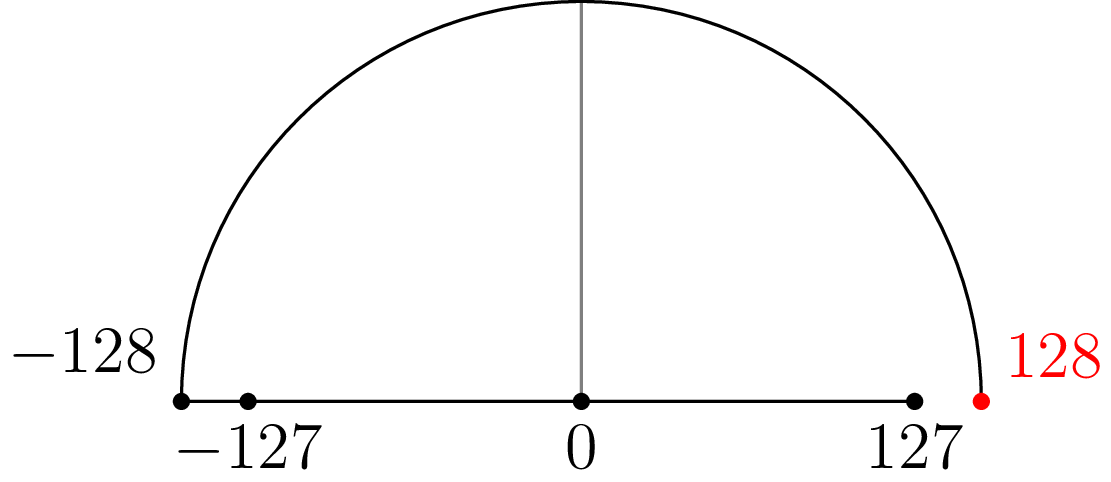Signed Integers are Asymmetrical

What’s wrong with this code?

int8_t absolute(int8_t x) {
if (x >= 0) {
return x;
} else {
return -x;
}
}

Seems straightforward enough. Let’s try it with some representative numbers:

#include <stdint.h>
#include <stdio.h>

int8_t absolute(int8_t x) {
if (x >= 0) {
return x;
} else {
return -x;
}
}

int main() {
int8_t values = {INT8_MIN, INT8_MIN + 1, 0, INT8_MAX - 1, INT8_MAX};
for (int i = 0; i < 5; i++) {
int8_t x = values[i];
printf("abs(%4i) = %4i\n", x, absolute(x));
}
return 0;
}

Running this code yields:

eudoxia@bullroarer \$ gcc cabs.c
eudoxia@bullroarer \$ ./a.out
abs(-128) = -128
abs(-127) =  127
abs(   0) =    0
abs( 126) =  126
abs( 127) =  127

The very first case is wrong. Why? Because signed integers are asymmetrical around zero. Note how INT8_MAX is 127, while INT8_MIN is -128. You can think of it in terms of a number line, with the negative side being larger by one:More generally: if a signed two’s complement number has n bits, the largest number it can represent is 2(n - 1) - 1, while the most negative number it can represent is -2(n - 1)

You can think of unary negation as rotating a number around zero on the number line. Evaluating -(-127) rotates the number and lands on 127:Evaluating -(-128) rotates the number around zero, but it lands one step beyond INT8_MAX. Because of overflow, it lands right back on INT8_MIN.Note that compiling with -ftrapv doesn’t help. Neither GCC nor Clang catch this. Ada does, though:

type Signed_Byte is new Integer range -128 .. 127;

function Absolute(X: Signed_Byte) return Signed_Byte is
begin
if X >= 0 then
return X;
else
return -X;
end if;
end Absolute;

type Index is range 1 .. 5;
type Value_Array is array (Index) of Signed_Byte;

Values: Value_Array := (127, 126, 0, -127, -128);

package Signed_Byte_IO is new Ada.Text_IO.Integer_IO (Signed_Byte);
begin
for I in Index loop
declare
X: Signed_Byte := Values(I);
begin
Signed_Byte_IO.Put(X);
Signed_Byte_IO.Put(Absolute(X));
end;
end loop;

Running this yields: